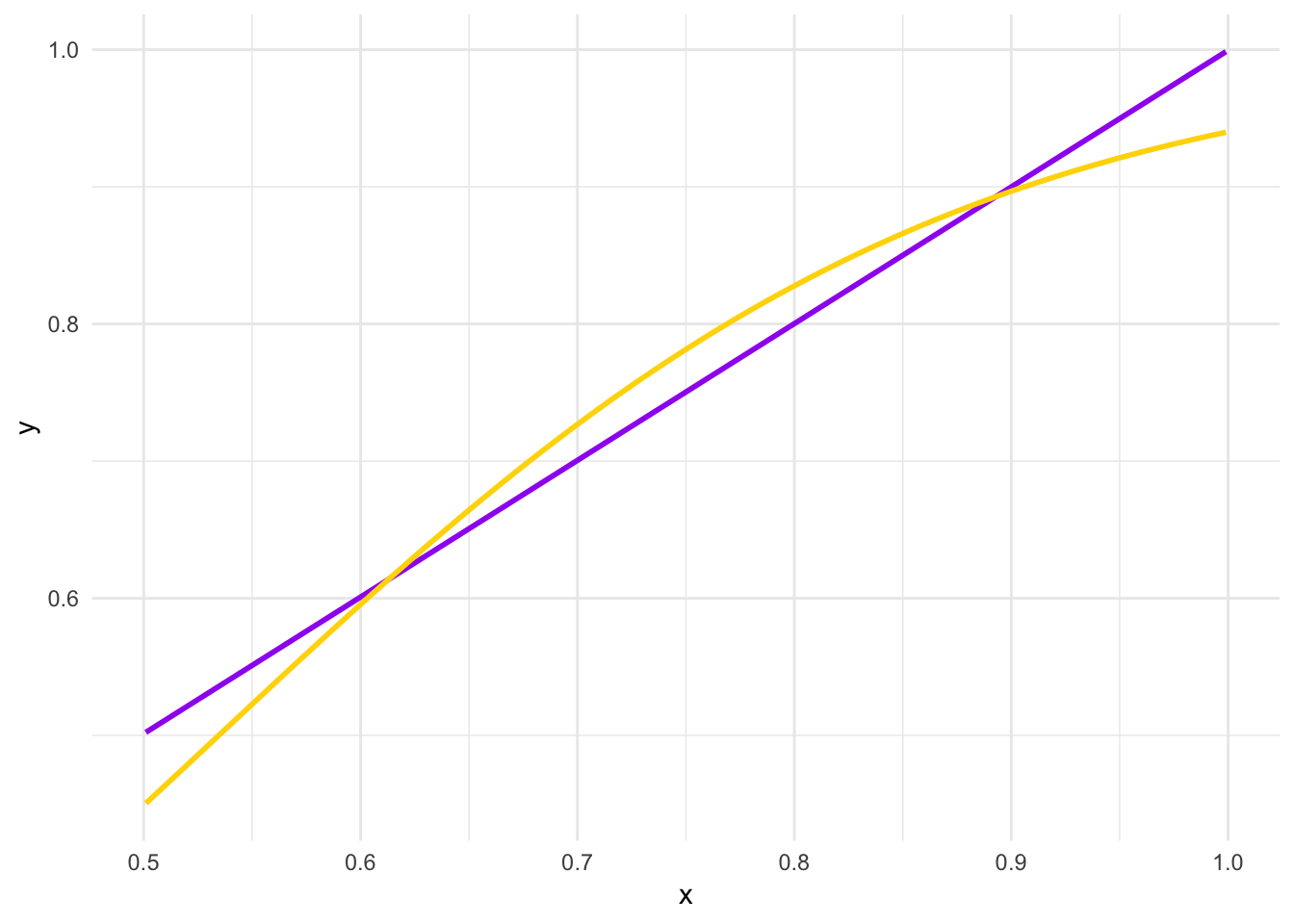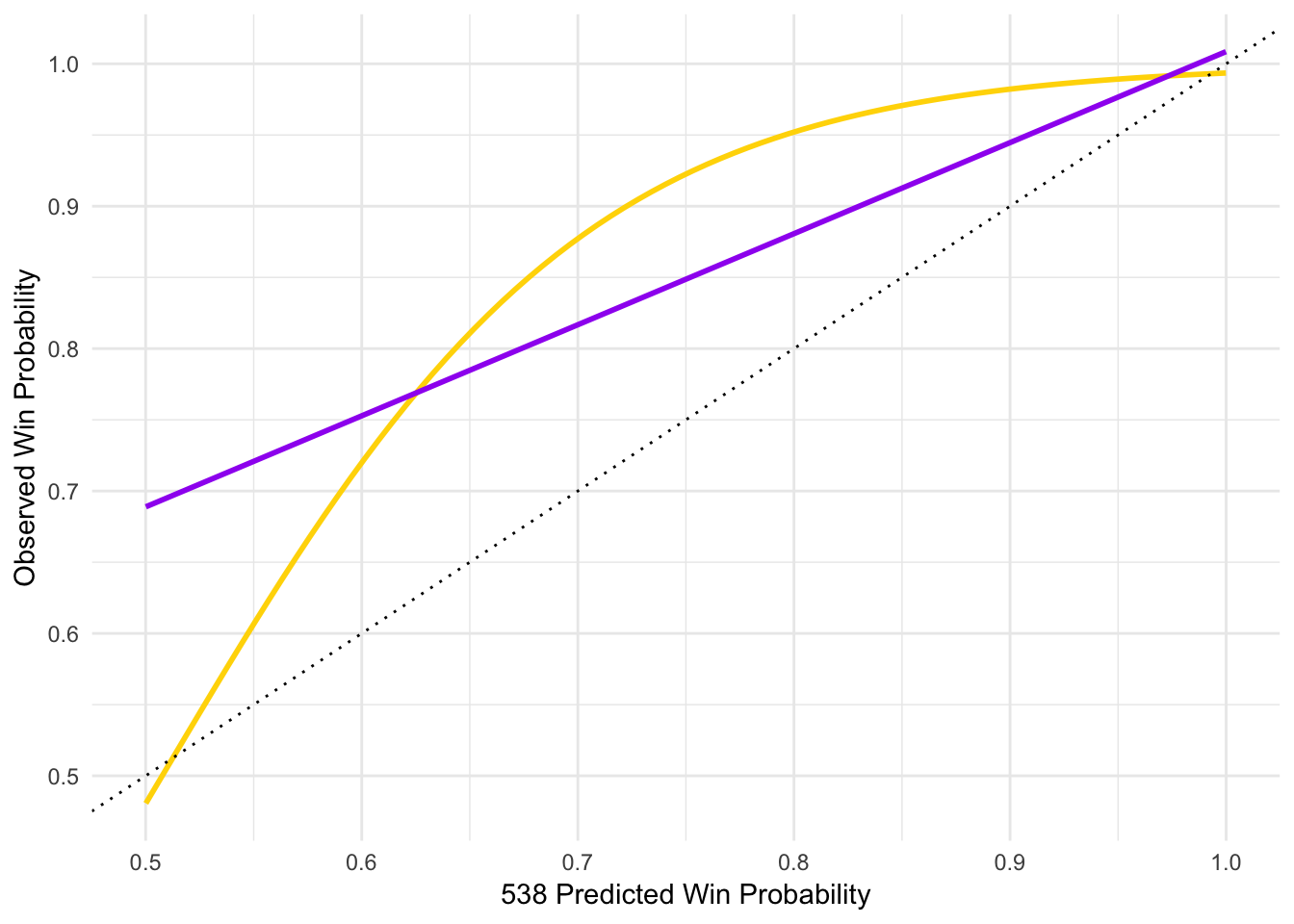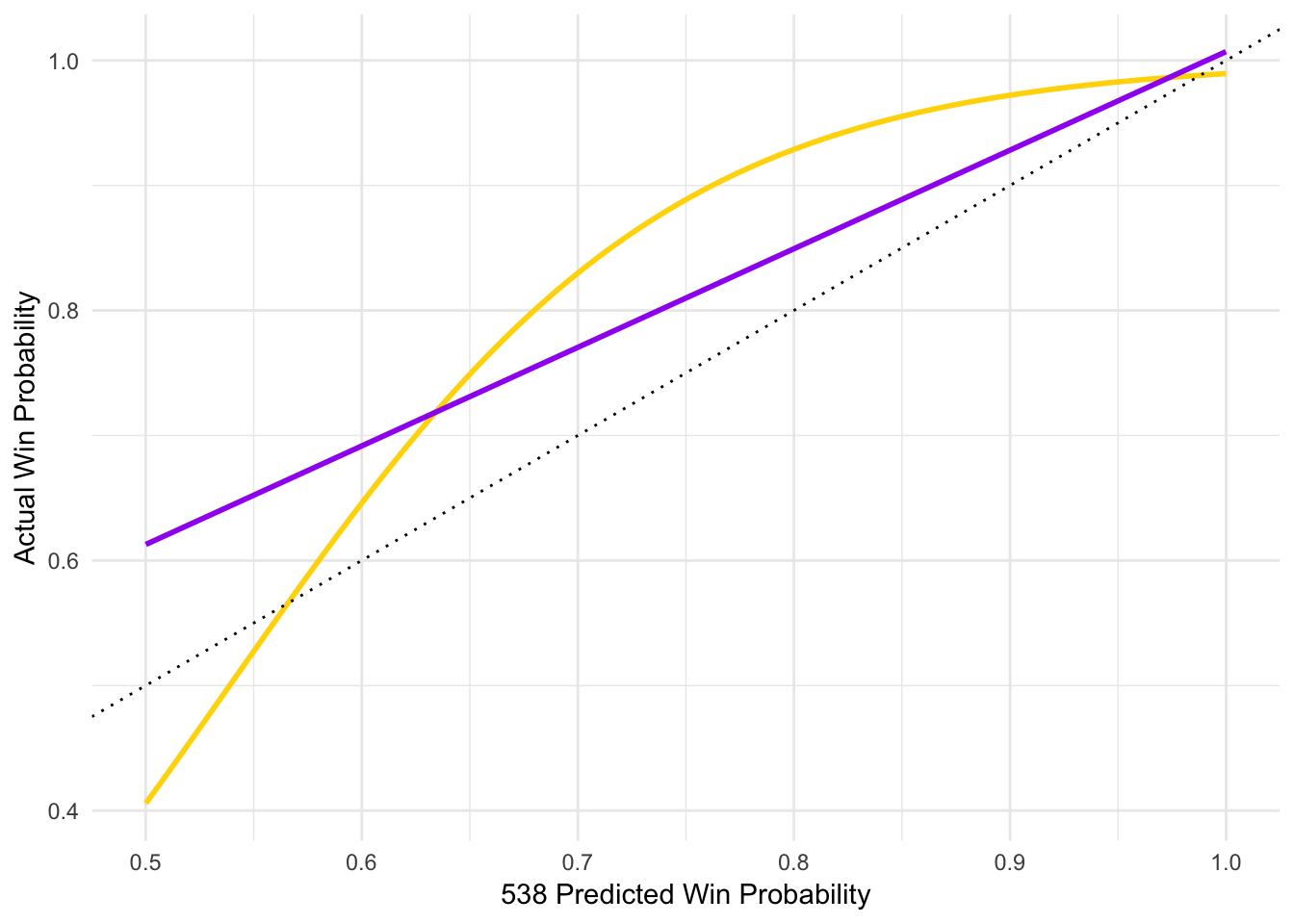How Do We Judge a Probabilistic Model? Or, How Did FiveThirtyEight Do in Forecasting the 2018 Midterms?

Nate Silver and the FiveThirtyEight folks talked quite a bit this election cycle about how their model was probabilistic. What this means is that they don’t just offer a single prediction (e.g., “Democrats will pick up 10 seats”); instead, their prediction is a distribution of possible outcomes (e.g., “We expect the result to be somewhere between Republicans picking up 5 seats to Democrats picking up 35”). One of the most common ways of thinking probabilistically is thinking in the long-term: “If this same election were to happen a large number of times, we would expect the Democrat to win 70% of the time.”

It is puzzling to judge one of these models, however, because we only see one realization of this estimated distribution of outcomes. So if something with only a 5% predicted chance of happening actually does happen, was it because (a) the model was wrong? or (b) the model was right, but we just observed a very rare event happening?

We need to judge the success of probabilistic models in a probabilistic way. This means that we need a large sample of predictions and actual results; unfortunately, this can only be done after the fact. However, it can help us think more about what works and who to trust in the future. Since FiveThirtyEight predicted 366 elections in the 2018 midterms, all using the same underlying model, we have the requisite sample size necessary to judge the model probabilistically.

How do we judge a model probabilistically? The model gives us both (a) predicted winners and (b) predicted probabilities that these predicted winners actually do win. The model can be seen as “accurate” to the extent that the predicted probabilities correspond to observed probabilities of winning. For example, we can say that the model is “probabilistically accurate” if predicted winners with a 65% chance of winning actually win 65% of the time, those with a 75% chance of winning actually win 75% of the time, etc.

I want to step away from asking if the model was black-and-white “right or wrong,” because—strictly speaking—no model is “right” or “correct” in the sense of mapping onto ground truth perfectly. The FiveThirtyEight model—just like any statistical or machine learning model—will make certain assumptions that are incorrect, not measure important variables, be subject to error, etc. We should instead ask if the model is useful. In my opinion, a model that makes accurate predictions while being honest with us about the uncertainty in its predictions is useful.

Was the FiveThirtyEight 2018 midterm model probabilistically accurate? Before we get to the model, I want to show what the results would look like in a perfect situation. We can then compare the FiveThirtyEight model to this “perfect model.”

I am going to simulate 100,000 races. Let’s imagine that we make a model, and this model gives us winners and predicted probabilities for each winner in every single race. What we want to do is model this predicted probability exactly. In my simulation below, the probabilities that actually generate the winner are the same exact probabilities that we pretend we “modeled.” This is called `x` below. These probabilities range from .501 to .999.

We then simulate the races with those probabilities. The results are called `y` below. The result is `1` if the predicted winner actually won, while the result is `0` if they lost.

``````set.seed(1839)
n_races <- 100000
x <- runif(n_races, .501, .999)
y <- rbinom(n_races, 1, x)
sim_dat <- data.frame(x, y)
``````##           x y
## 1 0.9215105 1
## 2 0.8136280 1
## 3 0.6239117 1
## 4 0.8290906 1
## 5 0.8180035 0
## 6 0.7973508 1``````

We can now plot the predicted probabilities on the x-axis and whether or not the prediction was correct on the y-axis. Points will only appear on the y-axis at 0 or 1; we would generally use a logistic regression for this, but that imposes a slight curve to the line. We know that it should perfectly straight, so I fit both an ordinary least squares line (purple) and logistic regression line (gold) below.

If the FiveThirtyEight model were completely accurate in its win probability prediction, we would expect the relationship between predicted win probability (`x`) and observed win probability (`y`) to look like:And this is what the results for the 2018 midterms (all 360 Senate, House, and Governor races that have been decided) actually looked like:I am using the deluxe forecast the morning of the election. The dotted line represents what would be a perfect fit. Any line that has a steeper slope and is mostly below the dotted line would mean that the model is overestimating its certainty; any line with a smaller slope and is mostly above the dotted line indicates that the model is underestimating its certainty. Since the line here resides above the dotted line, this means that the FiveThirtyEight model was less certain in its predictions than it should have been. For example, a race with a predicted probability of 60% winning actually turned out to be correct about 70-75% of the time.

However, there are a few outstanding races. As of publishing, these are:

State Race Type Candidate Party Incumbent Win Probability
MS Senate Cindy Hyde-Smith R False 0.707
GA House Rob Woodall R True 0.848
NY House Anthony Brindisi D False 0.604
NY House Chris Collins R True 0.797
TX House Will Hurd R True 0.792
UT House Ben McAdams D False 0.642

Let’s assume that all of these predictions come out to be wrong—that is, let’s assume the worst case scenario for FiveThirtyEight. The results would still look quite good for their model:We can look at some bucketed analyses instead of drawing a fitted line, too. The average FiveThirtyEight predicted probability of a correct prediction was 92.1%, and the average actual correct prediction rate was 95.8%. However, most of the races were easy to predict, so let’s limit these to ones that are below 75% sure, below 65% sure, and below 55% sure:

Threshold Count Mean Win Probability Correct Rate
0.75 45 0.627 0.756
0.65 30 0.591 0.667
0.55 8 0.522 0.750

The actual correct rate is higher than the predicted probability in all cases; again, we see that FiveThirtyEight underestimated the certainty they should have had in their predictions.

For a full view, here are all of the incorrect FiveThirtyEight predictions. They are sorted from highest win probability to lowest, so the table is ordered such that bigger upsets are at the top of the table.

State Race Type Candidate Party Incumbent Win Probability
OK House Steve Russell R True 0.934
SC House Katie Arrington R False 0.914
NY House Daniel Donovan R True 0.797
FL Governor Andrew Gillum D False 0.778
FL Senate Bill Nelson D True 0.732
KS House Paul Davis D False 0.641
IA Governor Fred Hubbell D False 0.636
OH Governor Richard Cordray D False 0.619
IN Senate Joe Donnelly D True 0.617
MN House Dan Feehan D False 0.599
GA House Karen C. Handel R True 0.594
VA House Scott Taylor R True 0.594
TX House John Culberson R True 0.553
NC House Dan McCready D False 0.550
TX House Pete Sessions R True 0.537

Is underestimating certainty—that is, overestimating uncertainty—a good thing? Nate Silver tongue-in-cheek bragged on his podcast that the model actually did too well. He was joking, but I think this is an important consideration: If a model is less certain than it should have been, what does that mean? I think most people would agree that it is better for a model to be 10% less certain than to be 10% more certain than it should have been, especially after the surprise of 2016. But if we are judging a model by asking if it is truthful in expressing uncertainty, then we should be critical of a model that makes correct predictions, but does so by saying it is very unsure. I don’t think there is a big enough discrepancy here to pick a fight with the FiveThirtyEight model, but I do think that inflating uncertainty can be a defense mechanism that modelers can use to protect themselves if their predictions are incorrect.

I think it is safe to say that FiveThirtyEight performed well this cycle. This also means that the polls, fundamentals, and expert ratings that underlie the model were accurate as well—at least in the aggregate. In my probabilistic opinion, we should not say that the FiveThirtyEight model was “wrong” about Steve Russell necessarily, because a predicted probability of 93% means that 7% of these will come out contrary to what was expected—and the observed probabilities mapped onto FiveThirtyEight’s predicted probabilities pretty accurately. That is, FiveThirtyEight predictions made with 93% certainty actually came to pass more than 95% of the time.

I think probabilistic forecasts are very useful for political decision makers. It keeps us from seeing a big deficit in the polls and writing off the race as unwinnable—we should never assume that the probability of an event is 100%. For example, it was said in the run-up to the midterms that a race needs to be close to even consider donating money to it: “If the race isn’t between 2% or 3%, you’re wasting your money.” There is uncertainty in all estimates—so candidates with a 90% likelihood of winning are going to lose 10% of the time. The trick for analysts and professionals are to make sense of probabilistic forecasts. Were there any warning signs ahead of time that hinted Steve Russell, Katie Arrington, or Daniel Donovan were going to lose? Was there a paucity of polling data are not a lot of money spent in those elections? Were there highly localized aspects to the races that were obscured by the model, which allowed for correlation between similar demographies and geographies? People should certainly invest more in races that are closer to being a toss-up, but thinking probabilistically requires is to invest some resources in longer shots than just a few percentage points, because unlikely events are unlikely, but they still do happen.

All data and code for this post can be found at my GitHub.